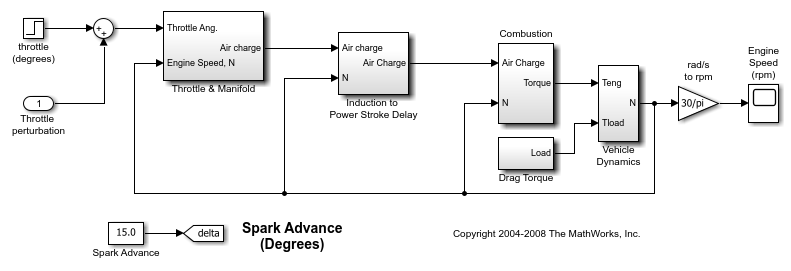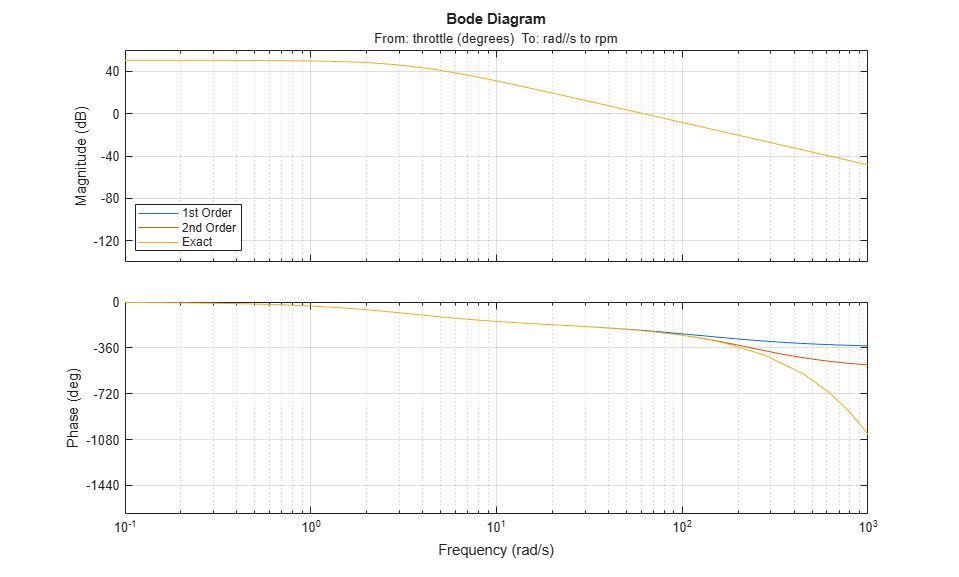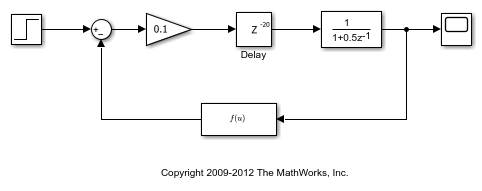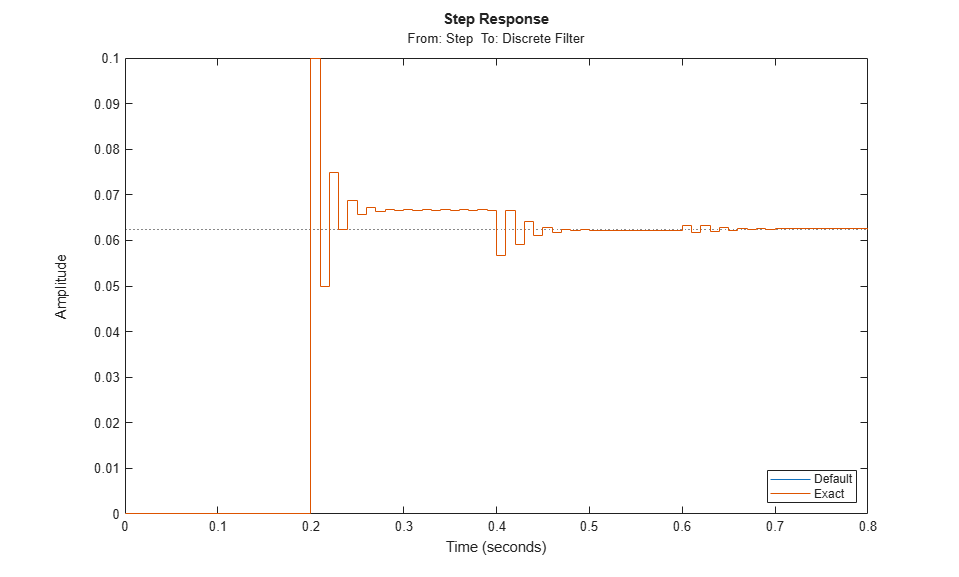# Linearize Models with Delays

This example shows how to linearize a Simulink® model that contains delays.

For more information on manipulating linearized models with delays, see Specifying Time Delays and Analyzing Control Systems with Delays.

### Linearize Model with Continuous Delays

You can linearize a Simulink model with continuous-time delay blocks such as the Transport Delay, Variable Transport Delay, and Variable Time Delay using one of the following options.

• Use Pade approximations of the delays to get a rational linear system through linearization. This option is the default method used by Simulink Control Design™ software.

• Compute a linearization where the delay is exactly represented. Use this option when you need accurate simulation and frequency responses from a linearized model and when assessing the accuracy of Pade approximation.

Open the engine speed model used in this example.

```model = 'scdspeed'; open_system(model) ```The Induction to Power Stroke Delay subsystem contains a Variable Transport Delay block named dM/dt. Specify the path to this block.

```DelayBlock = 'scdspeed/Induction to Power Stroke Delay/dM//dt delay'; ```

To compute a linearization using a first-order approximation, set the order of the Pade approximation to 1. For the Variable Transport Delay block, Pade Order property to `1`.

Alternatively, at the command line, enter the following code.

```set_param(DelayBlock,'PadeOrder','1'); ```

Specify the throttle angle as the linearization input and engine speed as the linearization output.

```io(1) = linio('scdspeed/throttle (degrees)',1,'input'); io(2) = linio('scdspeed/rad//s to rpm',1,'output'); ```

Linearize the model.

```sysOrder1 = linearize(model,io); ```

To linearize the model using a second-order approximation, set the Pade order to `2`.

```set_param(DelayBlock,'PadeOrder','2'); sysOrder2 = linearize(model,io); ```

To compute a linear model with the exact delay representation, create a linearization options object and enable the `UseExactDelayModel` option.

```opt = linearizeOptions; opt.UseExactDelayModel = 'on'; ```

Linearize the model using the specified linearization options.

```sysExactDiscrete = linearize(model,io,opt); ```

Compare the Bode response of the Pade approximation models and the exact linearization model.

```p = bodeoptions('cstprefs'); p.Grid = 'on'; p.PhaseMatching = 'on'; p.XLimMode = {'Manual'}; p.XLim = {[0.1 1000]}; bode(sysOrder1,sysOrder2,sysExactDiscrete,p); legend('1st Order','2nd Order','Exact','Location','SouthWest') ```In the case of a first order approximation, the phase begins to diverge around 50 rad/s and diverges around 100 rad/s.

```bdclose(model) ```

### Linearize Model with Discrete Delays

When linearizing a model with discrete delay blocks, such as (Integer) Delay and Unit Delay blocks, use the exact delay option to account for the delays without adding states to the model dynamics. Explicitly accounting for these delays improves simulation performance for systems with many discrete delays because there are fewer states in your model.

Open a Simulink model of a discrete system that contains a Delay block with 20 delay states.

```model = 'scdintegerdelay'; open_system(model) ```By default the linearization includes all of the states folded into the linear model. Set the linearization input and output signals and linearize the model.

```io(1) = linio('scdintegerdelay/Step',1,'input'); io(2) = linio('scdintegerdelay/Discrete Filter',1,'output'); sysDefault = linearize(model,io); ```

View the model size. It has 21 states (1 - Discrete Filter, 20 - Integer Delay).

```size(sysDefault) ```
```State-space model with 1 outputs, 1 inputs, and 21 states. ```

Linearize the model using exact delay representation.

```opt = linearizeOptions; opt.UseExactDelayModel = 'on'; sysExactDiscrete = linearize(model,io,opt); ```

View the resulting model size. It has 1 state. The delays are accounted for internally in the linearized model.

```size(sysExactDiscrete) ```
```State-space model with 1 outputs, 1 inputs, and 1 states. ```

Compare the performance of the models using a step response. The models produce the same response.

```step(sysDefault,sysExactDiscrete) legend('Default','Exact','Location','SouthEast') ``````bdclose(model) ```